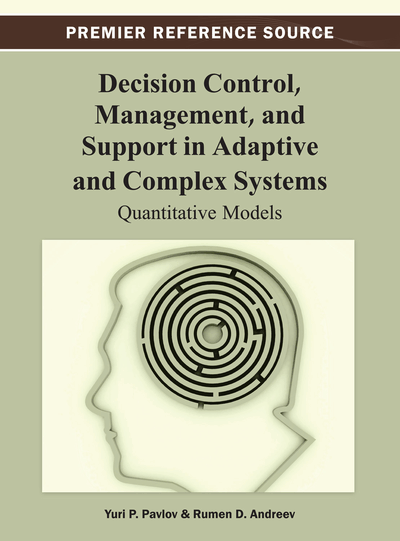# Preferences-Based Performance Measurement Models

DOI: 10.4018/978-1-4666-2967-7.ch003

## Abstract

It is shown that the human subjective expectations for the uncertainty events can be described mathematically with the terms of the probability theory and can be inserted into the mathematical theory of von Neumann and Morgenstern. Some examples of utility functions are shown.
Chapter Preview
Top

## 1. Theory Of Measurement And Some Basic Measurement Scales

In the current chapter, we consider the term measurement from a general point of view as we consider necessary notions and mathematical formulations in the framework of these general settings. In a broader plan, the scientific progress is a constant development and refinement of the terms and their mutual relations, with regard to known or learned facts from the objective reality. The mathematical description on such a fundamental level requires basic mathematical terms, as sets, relations, and operations over them, as their gradual elaboration to more complex and specific terms as functions, operators on mathematically structured sets as well, as equivalency of these descriptions with respect to a given real object (Pfanzagl, 1971). In the last aspect of equivalency of the mathematical descriptions, we enter the theory of measurements and scaling (Krantz, 1971, 1989, 1990).

Generally speaking, we can divide the measurements in two basic types, fundamental and derivative from them. The fundamental measurements are in the first stage of the scientific investigation and development, in which several real manifestations formed as new terms are subject to estimation. The derivative measurements appear on the next stages after the basic terms have been well investigated and measured (metrified). These new measures are constructed based on the several basic fundamental scales of metrizations in the investigated scientific domain. We can say that in some sense the theory of measurement and scaling considers the mathematical description of the way of forming the mathematical metrization of the fundamental terms. Of course, the investigations in the real life hardly ever follow the described in such a way the consecutive ways of scientific branches formation.

We start with defining the term relation (Pfanzagl, 1971). Let А={x1, x2, x3,....,xn, ....} be a set of elements (different occurrences of the fundamental terms which are in the base of the investigated phenomenon). This set, in general setting, can be be infinite, countable or of greater power. The set of all pairs (x1, x2) forms the Cartesian product А×А, such that x1А and x2А. Every subset of this set is called binary relation. It is possible to consider ternary, quaternary or m-ary relations defined over the set А×А×А…А×А×А or in other terms Аm is the m-ary Cartesian product of the set А. Further, we will mostly consider binary relations. Shortly we are going to consider the binary relation as an ordered pair (А, R), and the belonging of the ordered pair (x1, x2) to R will be denoted by x1Rx2 or R(x1, x2)=1. For the m-ary relation the notation is (x1, x2, x3, ....,xm)R or R(x1, x2, x3, ....,xm)=1. We are going to mention some of the basic properties of the binary relations, necessary for the further description.

We start with the term reflexivity (Pfanzagl, 1971; Dixmier, 1967). The relation R is reflexive if the main diagonal belongs to the subset R, (x,x)R,x∈А. We get irreflexivity when the main diagonal does not belong to R.

The binary relation R is symmetric, if from (x, y)R follows (y, x)R.Asymmetric is when from (x, y)R follows (y, x)R.

## Complete Chapter List

Search this Book:
Reset